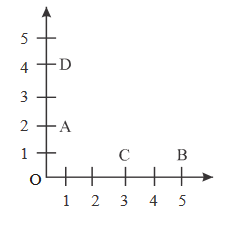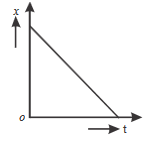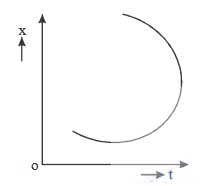11.

Here is a cube made from twelve wire each of length l. An ant goes from A to G through path A-B-C-G. Calculate the displacement.A. $$2l$$

B. $$3l$$

C. $$\sqrt{3}l$$

D. $$\frac{l}{\sqrt{3}}$$

12.

As shown in the figure particle P moves from A to B and particle Q moves from C to D. Desplacements for P and Q are x and y respectivey thenA. $$x > y$$

B. $$x < y$$

C. $$x = y$$

D. $$x\geq y$$

13.

Shape of the graph of position $$\rightarrow$$ time given in the figure for a body shows thatA. The body moves with constant acceleration

B. The body moves with zero velocity

C. The body returns back towards the origin

D. Nothing can be said

14.

The graph of position $$\rightarrow$$ time shown in the figure for a particle is not possible becauseA. Velocity can not have two values on one time

B. Displacement can not have two values at one time

C. Acceleration can not have two values at one time

D. A, B and C are true

15.

An ant goes from P to Q on a circular path in 20 second Radius OP = 10m. What is the average speed and average velocity of it ?A. $$\frac{\pi }{6}ms^{-1},\ 3ms^{-1}$$

B. $$\frac{\pi }{3}ms^{-1},\ \frac{\sqrt{3}}{2}ms^{-1}$$

C. $$\frac{\pi }{3}ms^{-1},\ \sqrt{3}ms^{-1}$$

D. $$\pi ms^{-1},\ \sqrt{6}ms^{-1}$$

16.  A particle is thrown in upward direction with initial velocity of 60 m/s. Find average speed and average velocity after 10 seconds. [$$g=10\ ms^{-2}$$]

A. 26 m/s, 16 m/s

B. 26 m/s, 10 m/s

C. 20 m/s, 10 m/s

D. 15 m/s, 25 m/s

17.  The ratio of path length and the respective time interval is

A. Mean Velocity

B. Mean Speed

C. Instantaneous Velocity

D. Instantaneous Speed

18.  A car moving over a straight path covers a distance x with constant speed 10 m/s and then the same distance with constant speed of $$V_{2}$$. If average speed of the car is 16 m/s, then $$V_{2}$$ = ?

A. 30 m/s

B. 20 m/s

C. 40 m/s

D. 25 m/s

Explanation :

Average speed $$=\frac{2V_{1}V_{2}}{V_{1}+V_{2}}$$

19.  A bus travels between two points A ans B. $$v_{1}$$ and $$v_{2}$$ are it's average speed and average velocity then

A. $$v_{1} > v_{2}$$

B. $$v_{1} < v_{2}$$

C. $$v_{1} = v_{2}$$

D. Depends on situation

20.  A car covers one third part of its straight path with speed $$v_{1}$$ and the rest with speed $$v_{2}$$. What is its average speed ?
A. $$\frac{3v_{1}v_{2}}{2v_{1}+v_{2}}$$
B. $$\frac{2v_{1}v_{2}}{3v_{1}+v_{2}}$$
C. $$\frac{3v_{1}v_{2}}{v_{1}+2v_{2}}$$
D. $$\frac{3v_{1}v_{2}}{2v_{1}+2v_{2}}$$